Chapter 0.B Backgrounds for Calculus (draft)
August, 2006: This page now requires Internet Explorer 6+MathPlayer or Mozilla/Firefox/Netscape 7+.
2. Functions Introduction and Review.

Preface: There are many different kinds of numbers and ways to express, compare, and relate them. Wherever we turn, whatever we read, we find numbers used to describe situations while the relations between numbers help us understand, plan, and control these situations.  The special kind of relation between numbers that is called a function, first recognized explicitly by Leibniz, evolved through the work of the Swiss mathematician Leonhard Euler (1707-1783)  ( pronounced "Oiler") and the French mathematician Joseph Lagrange (1763-1813) to become a cornerstone for mathematics in the 20th century. Though Newton, Leibniz and the other early developers of the calculus did not have the current concept of a function to assist them, we would handicap ourselves seriously if we did not recognize and use the function concept today.

Most students in a precalculus course have had some experience with functions in their previous studies. A complete and detailed introduction and review of function concepts and examples early in this course would delay our reaching the object of our studies, the analysis of the functional relations with calculus course. For this reason, our review in this section will be limited to an exploration of the basic nature of functions and ways to think about functions that make sense for describing, visualizing and analyzing them. Thus our attention is directed primarily at functions and change.  You will find a catalog of definitions and fundamental properties of functions in Appendix ***.

The Trip: One simple and very useful context for understanding functions is that of a car traveling on a road, what we might call "the trip." Numbers that are interesting here are often found on the dashboard of the car and by the roadside. They measure such quantities as the amount of gas in the gas tank, the distance traveled during this trip, the distance traveled by the car since it was "new," the temperature of the engine, the mileage markers on the side of the road, the speed limit markers, the speedometer giving the car's speed, the tachometer indicating the rate at which the engine is turning, and the clock.

For sure when we are considering any trip in a car we are aware that time is changing whether the car is standing still or moving very quickly. Thinking about the relations between these quantities can help us gain some informal understanding of functions and how they can be used to understand the trip. For example, the distance the car has traveled is related to the gas the car's engine has consumed. The temperature of the engine is related to the rate at which the engine is turning. All  the measurements can be thought of as related to time, since when we make a measurement we do that at a particular time.

The concept of function can be applied in many contexts beyond that of real numbers. For the purpose of beginning our study of functions, we will use the concept only for examining functions that relate real numbers. These functions are usually described as real valued functions of a real variable. Other function concepts studied in mathematics treat relations between three or more variable numerical quantities as well as variables that are not numbers but geometric points and vectors.

Static Functions as Relations: The mathematical concept of function connects quantities while suggesting informally something stronger than just a relation between the quantities. In one sense when we describe a function relationship between variable  quantities, we are establishing a priority or an order to the information. When two variables are related we often can identify one as being an independent, governing, or controlling variable while describing the other as a dependent, regulated, or controlled variable. These descriptives indicate an important quality of a function, namely that knowledge, assignment, or specification of one variable's value will determine the value of the other variable.  In this sense we can consider each of the variables in the trip context as functions controlled by the time variable. It is not that there is any necessary scientific or causal relation between time and the position of the car (or any of the car variables). The function language merely indicates that knowing the time should allow us to determine the position of the car.
A function connects the information about the variables by pairing the data and assigning a priority to the pairs.
Knowing the first number of a pair uniquely determines the pair, and thus the second number of the pair.

 Dynamic Functions - paired changes. There is a second way to think of functions relating variables. We consider the function as a mechanism or interpreter that transforms one measurement or number into a second number. [It helps to avoid thinking of this process as being strictly causal in physical situations, even though there is some strong connection to causality with a priority to the order of the relation.] In the trip context we can think of the car dashboard as a mechanism. Knowing the time by reading the clock allows us to determine what the reading on the odometer is. The distance our car has traveled is a function of time. One can also connect the amount of gas in the gas tank, measured by the gas gauge, to the distance the car has traveled during a trip, thus the amount of gas is a function of the distance traveled. The history of clocks and watches and the measurement of time are a fascinating part of the development of science and mathematics. Time certainly plays an important role in our lives today, one that we sometimes overlook too easily. We take for granted our ability to measure time with precision to the microsecond, but in 1773 the British government paid a 20,000 pound sterling prize to John Harrison for developing a very accurate navigation chronometer with which to calculate longitude.

This mechanistic view of functions goes particularly well with the use of calculators.  You enter a number on the display, push a single button and the (resulting) number on the display is usually changed.

The terms "input" and "output" are used here to describe the values of the controlling and the controlled variables respectively. When  thought of as a machine, a function will process some numbers while being unable to process others. For example, if the process returns the multiplicative inverse (reciprocal) of a number, then the process will work well on all numbers with the single exception of 0. Or if the process returns the real number square root of a given number, then the process will operate on non negative real numbers but will fail to return a result for negative real numbers. [Remember, the square root of a negative number is not a real number, but an imaginary number.]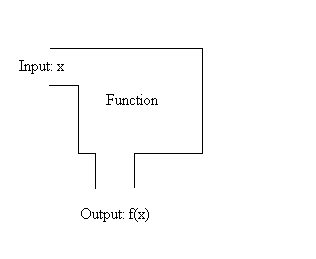We refer to the collection of numbers which the function can process as the source or domain of the function. Thus the function that returns the reciprocal of a number has a source of the set of all non zero real numbers, while the source for the real valued square root function is the set of non negative real numbers. The source of a function when not described explicitly is assumed to contain all the inputs that work.

It is useful to describe the source of a function so you can avoid errors that sometimes arise from applying the function in meaningless situations. The most common algebraic restrictions on the source of a function arise from division by 0 and finding square roots of negative numbers.
For example, the function which returns the number `1/{1-x^2}` for an input of the number x can have a source that includes all real numbers except 1 and -1.
As a second example, the function that returns the number `sqrt{1-x^2}` for the input number x can have a source that includes only numbers in the interval [-1,1].

Notation for Describing Functions: As these last examples demonstrate, it would be convenient to have a notation that allows us to describe a function either from the static view of a collection of ordered pairs or from the dynamic view by designating what the function yields as output for a specified, yet arbitrary, input. The notation and vocabulary that has evolved (and is still evolving) must distinguish at least three things: the first number of the pair, called the argument or the input number, the second number of the pair, called the result, value, or the output number, and the designation or name for the function.

 Variable Relations: A traditional approach to this notation considers 2 variable names, say x and y, with x representing the first number for the function and y representing the second number. In this approach there is no name for the function, only a statement that y is a function of x and an equation describing an explicit relation between the variables, such as y = 2x+3,  or an implicit relation such as `x = y^2`  and `y>=0`. This approach abuses the notation since the letter y refers both to the number and the function relation of that number to the number x. For example, when the function y is described by the equation `y = 2x+3`  we can determine the value of `y ` when `x = 6` by substituting 6 for `x` in the formula `2x+3` to find `y = 2 (6)+3=15`. The ordered pairs determined by this function have the form `(x,y)` where `y=2x+3` or `(x,2x+3)`. For `x = 6`, the information from the function is that (6,15) is one of the pairs of the function.  The evaluation of y when x=6 has a traditional notation of `y| _{x=6}=``  2x+3| _{x=6}=2(6)+3=15` Beware: A common error is confuse the parentheses in this notation for the value of a function  for the parentheses used frequently to collect terms in an expression to be treated as a single number in some algebraic calculation like multiplication.  By convention, when f is a function, the symbols `f(t+5)`  means the value of f  for the number described by `t+5`, it does not mean the product of the number `f` by the number `(t+5)`.

Function Values: A more contemporary approach to the notation (based on the notation used by Lagrange in his work at the end of the 18th century), with which you are no doubt somewhat familiar, assigns to the function a specific letter or symbol  that suggests what the function does or at least gives the function a recognizable name.

For example, the function might be the square root function. The square root function is assigned the shorthand name sqr, or, as with less familiar functions, it might be named temporarily for the purpose of discussion with the symbol f or g. Once the name has been given, the relation between input and output, argument and result, can be described in a number of ways. A variable name is given, or presumed, for the input, like x or t, and then the output is described as the value of the function at x or t, which is denoted most often as f(x) or f(t).  Notice that this last notation for the value of the function at x has four symbols: f denotes the name of the function, x denotes the name of the number to be input, the two parentheses separate the name of the function from the name of the variable.

A description of the function can then be accomplished by giving a  procedure for finding the value of `f(x)`, such as `f(x)=2x+3`. Once `f`  has been described and thus defined for the purpose of discussion, the notation allows us to denote the value of the function  that corresponds to the number 6 as `f(6)`. This number can be computed using the defining equation: `f(6)=2(6)+3=15`. Likewise the value of the function for the number `-4` is denoted `f(-4)`, and can be computed to  be `f(-4)=2(-4)+3=-5`. For the expression `t+5`, which can represent a number, the value of the function is denoted `f(t+5)`, which can be simplified by using the definition of `f` so that `f(t+5)=2(t+5)+3 = 2t+13`.

The function value notation is sometimes combined with the two variable notation in statements such as "suppose `y` is a function of `x`, with `y=f(x)=2x+3`." Then evaluation can be expressed by the equation: `y| _{x=6}=f(6)=2(6)+3=15`.

Transformation Notation: Another current approach to describing the input/output information of a function is to give the name of the function, then a name for an input variable followed by an arrow and an expression describing the output that results from the input. For example, `f : x -> 2x+3` or more generally  `f : x -> y = f(x)`.

The arrow in the notation helps convey visually the dynamic aspect of a function that transforms a number. This notation helps underscore the active nature of those functions that in some way do require a construction of the resulting number values by some conceptual (and perhaps even mechanical or electronic) process.

Multi-case functions: In some contexts a function cannot be described by a single simple algebraic formula using well known conventional functions. This can be for many reasons:
• There may be no algebraic formula that captures that the relation;
• The function may arise from a non algebraic context;
• The function may piece together some more simple function.

To piece together simple functions, the most common method is to establish some tests on the source numbers. These tests determine precisely what the appropriate method is for determining the function's value.

Here is an example of a function F defined by cases.

• Case 1: if `x <= 0`, then `F(x) = 0`;
• Case 2: if  `0< x <4` then `F(x) = {x^2}/16`, and
• Case 3: if `4 <= x` then `F(x)=1`.
• To use this definition of `F` we need to first check under which case the argument  for evaluation falls, then follow the function's rule as appropriate.
So, to find `F(-3)` we see first that `-3` falls under Case 1, so `F(-3) = 0`.
To find `F(3)` we check that `3` is under Case 2, so `F(3) = {3^2}/16 = 9/16`.
Finally to find `F(5)` we use Case 3, so `F(5) = 1`.

The conventional notation for this function's definition is expressed by a multi-lined equation: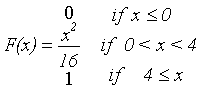Functions and Tables: In some cases we do not know any formula that precisely matches the pairings for a function. This is in fact  mot common when we look at any function that arises from real world phenomena.  We may have a formula that provides a good estimate or we have only some data . Sometimes this data is measured and recorded at selected numbers (or times). In this case the information we have for the function may be displayed in the form of a table.

For example if f is the function recording the room temperature we may know only that f(0) = 60, f(4) = 55, f(8) = 65, f(12) = 68, f(16) = 68, f(20) = 68, f(24) = 60. It is convenient to put this data in a table. See Table 1.

Be careful not to assume too much about a function that is represented only by a table of values at selected numbers. For example, although  f(0)= 60 and f(4)=55, you cannot assume that f(2) is between 60 and 55.
 x f(x) 0 60 4 55 8 65 12 68 16 68 20 68 24 60
Table 1

Non algebraic [Geometric] Functions: If we think of the room temperature function again, we can imagine a device recording the temperature  graphically with a sheet of paper moving at a steady rate under a marking pen attached to a temperature sensitive device that moves the pen depending on the room temperature. This would give a rather different record of the room's temperature as a function of time. Once scales are established on the paper for the time and temperature, we can give a better estimate of the temperature at many different times by being able to read the graph. This graphical presentation of a function gives much more information than a table, but you should be careful here as well not to infer too much from the graph, especially since the mechanism, the recording instrument, and the scales all can contribute to the imprecision of the information.

Curves and coordinates in Cartesian geometry. Using numbers to study figures in geometry is not a very recent part of mathematics. And using figures to study connections between numbers is also not new to math. In your previous course work you have studied the algebraic relations between coordinates of points on lines, circles, and other curves and conversely have graphed figures to illustrate the relation between numbers involved in an equation. The key in these correspondences has been the connection usually attributed to Rene Descartes in analyzing curves with numbers through use of common measurements and variables related by equations determined by the geometry, called analytic (or Cartesian) geometry.

Coordinate system analysis applied to planar curves can lead to function relations that are commonly encountered in science. Measuring devices record the changes during some experiment as a curve. The variables connected to the curve are associated with the experiment using rectangular coordinates and then the curve is interpreted as representing the relation between these variables. Without any prior knowledge of the relation of the variables, neither can be assumed to be controlling, but there is a general convention to consider the horizontal, first coordinate variable (X) as controlling with the vertical and second coordinate (Y) as the controlled variable. But for a curve to give a function relation between these variable there needs to be more either understood implicitly or made explicit.

In particular, when given a value for X we need a way to determine a value for the Y variable using the curve as the mechanism for that determination. This is easy enough in many familiar cases. For the given value of X, say a, find a point P on the curve with that value as its first coordinate, i.e., P has coordinates (a,b) for some number b. When there is only one point P with first coordinate a on the curve, then the value b is uniquely determined by the curve, and b is the value of the Y variable corresponding to a. In this case we say that the curve has determined Y as an explicit function of X  and assume we are using the coordinate convention just described.

The Slope of the Tangent to a Curve as a Function: There are other functions we can associate with curves in analytic geometry. For many curves we can determine at each point on the curve a line that very close to the point looks indistinguishable from the curve and yet close to the point meets the curve only once. As mentioned in section 0.A, these lines are sometimes referred to as touching or tangent to the curve at the point, or tangent lines. To repeat the example from 0.A, to find the tangent line to a circle at a point P, you need only draw a radius from the center of the circle to the point P and then construct the line perpendicular to the radius at P, which by Euclidean geometry must be the tangent line. So how does this give rise to a function using numbers?

Consider the case when the curve determines Y as an explicit function of X. For a given value of X, say a,  we again find a unique point P on the curve with that value as its first coordinate, i.e., P has coordinates (a,b) for a unique number b. Now it sometimes turns out that we can find a unique, non vertical tangent line to the curve at P and determine the slope of this line, which we will call m. Since the value of m is uniquely determined using the curve from the value of X, m is a function of X. The value of m is derived geometrically from the original curve using the measurement of the slope of the tangent line at the point P determined by a.

Area of Geometric Figures as Functions: One of the most frequently encountered problems in geometry is that of finding a general method for determining the area enclosed by a class of planar figures. You have learned formulae for areas of squares, rectangles, triangles, trapezoids, circles, and perhaps two or three other general shapes. The measurement of these areas is usually based on other measured features of the figures, such as lengths of sides or relevant line segments and sometimes even the size of angles.

The relationships between area and these other variables of geometric figures can often be described as functions. For example we can consider rectangles that have a base of length 20 centimeters and determine the area when the altitude has length l centimeters. Not too hard.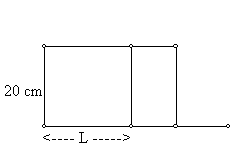The area is 20 l square centimeters. Or in the same setup, we can determine the length of the altitude when the rectangle has area A square centimeters with almost as little effort to be `A/20` centimeters. It requires a little more thought to determine the length of the diagonal of the rectangle with this setup based on the area A to be `sqrt{A^2+1600}/20`.
Another familiar area relation is found in the circle where the equation  `A=pir^2` allows us to determine either the area or the radius of a circle by knowing the other measurement. A slightly more subtle area relation was described in section 0.A. As the example there demonstrated there are many ways to measure a region in the plane, like a triangle, and passing a line across that region can give an area function determined by the position of the line.

Implicit functions: Let's consider the equation 3X + 4Y = 24 where X and Y represent real numbers. There are many possible choices for X and Y,  some of which will make the equation true (say X = 4 and Y = 3 ) and some of which will not (say X = 1 and Y = 2). Given a value for X there is one and only  one value for Y which will make the equation true. Thus if X = 1 then for the equation to be true we have 3 + 4Y = 24 , so 4Y = 21 and Y = 5.25. Since the equation determines a unique value for Y from any choice of X, we can say that the equation has determined Y implicitly as a function of X.
A more subtle relation is presented by the equation  X2 + Y2 = 25. This equation can be satisfied by many functions. For example `f(x)=sqrt{25-x^2}`, `g(x)=-sqrt(25-x^2)`, or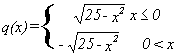. Here the equation does not determine a single value of one variable from the choice of another value but allows many possible functions which will satisfy the equation when the Y is determined by one of these functions. In this case the functions are described as being defined implicitly by the equation.

 Comment: With only a limited list of function values there is no way to tell what the function might have for its graph  without assuming some more restrictive qualities. For example, suppose the graph of the function is known to pass through two points with coordinates (0,0) and (1,1). You might think this is the function with `f(x) = x`, but in fact it could be `f(x)=x^2` or `f(x) = x^3` or `f(x) = x^2 + x - x^3`.  In fact if we consider all functions with f(0)= 0  and separately those functions with f(1)=1, we are only asking for those functions that satisfy both conditions, which doesn't seem like a large restriction when we think of all the different ways we could connect the points with coordinates (0,0) and (1,1) in a Cartesian plane.

Visualizing Functions and Transformation Figures: The key idea in visualizing functions with mapping diagrams or transformation figures is to have two parallel number lines representing the source (domain) and the target (range). The function can be thought of as a process relating points (numbers) on these two lines.

A point element on the source line is chosen which corresponds to a number. The function is applied to that number, and the resulting value is found represented on the target line. An arrow drawn from the point on the source line to the corresponding point on the target line visualizes the relation between the corresponding numbers.

In one sense, the transformation figure is a visualization of a function table. The numbers in the two columns of the table are represented by points on the two lines in the figure. The function relation that the table displays implicitly by having corresponding numbers in the same row is visualized in the figure by the arrow.  While the relative size of the numbers in the target column of the table is not represented in the display, the transformation figure uses the number line order to represent this aspect of the function's values.

Here is an illustration that should help you see some of these features. You can work on other examples after this one to begin to see some of the power of this visualization. [ To see a dynamic example of a transformation figure for linear functions, follow this link.]

Example: Suppose f(x)=2x+ 3. Table 1 shows a selection of the values this function relates, while this same information is visualized in Figure 4. Notice that larger numbers in the source column of the table correspond to larger values in the target column. On the transformation figure this feature can be seen by the fact that the lines connecting the corresponding points on the source and target lines do not cross. This is evidence of a function with increasing values.
 x f(x)=2x+3 5 13 4 11 3 9 2 7 1 5 0 3 -1 1 -2 -1 -3 -3 -4 -5 -5 -7
Table 1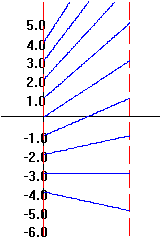Figure 4

Graphs of Functions and Other Relations: In  your previous work with functions and equations you have worked extensively with the graphical visualization using Cartesian coordinates for the plane to identify the function pairing of numbers.
In the graph of a function f we identify the pair of numbers a and f(a) with the point in the plane with coordinates (a,f(a)). We can plot marks at many of these points but when the domain of the function is an interval or as is more common all real numbers, we cannot hope to plot all the points. Instead we try to give a sense of how the points are related by drawing a curve that passes through some points that are known to be on the graph of the function. In doing this we are drawing figures much as students in elementary school draw figures by connecting the dots in order, or as economists graph the hour to hour price of some stock on the stock market or as a chemist would visualize the minute by minute temperature reading on a laboratory thermometer during an experiment.
Here are some examples of transformation figures and graphs. On the left are the tables of values for the functions at selected points, while on the right are the corresponding figures and graphs. [Graphs and Figures made using Winplot.]
Example 0.B.2  f(x) = x
 x f(x)=x 2 2 1 1 0 0 -1 -1 -2 -2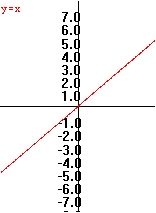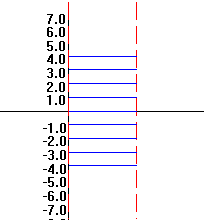Example 0.B.3   f(x) = -x

 x f(x)=-x 2 -2 1 -1 0 0 -1 1 -2 2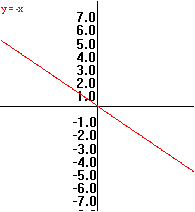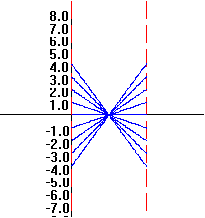Example 0.B.4   f(x) = |x|

 x f(x)=|x| 2 2 1 1 0 0 -1 1 -2 2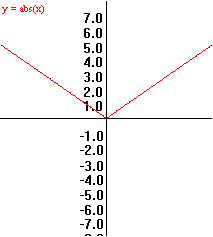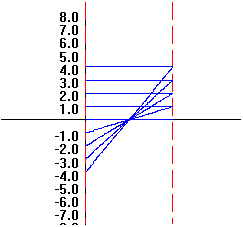Example 0.B.5  f(x) = 2x

 x f(x)=2x 2 4 1 2 0 0 -1 -2 -2 -4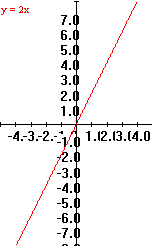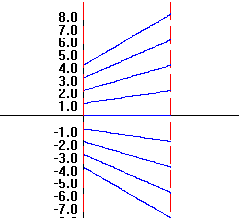Example 0.B.6  f(x) = x+1

 x f(x)=x+1 2 3 1 2 0 1 -1 0 -2 -1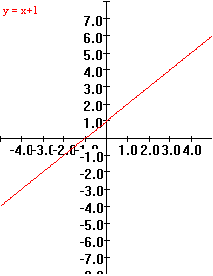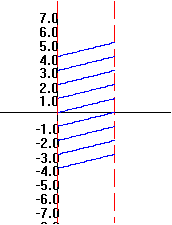Example 0.B.7  f(x) = x 2

 x f(x)=x 2 2 4 1 1 0 0 -1 1 -2 4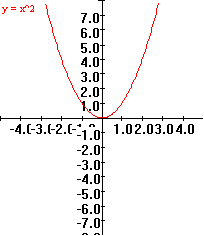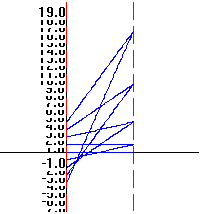Example 0.B.8  f(x) = 1/x

 x f(x)=1/x 2 0.5 1 1 0 ?? -1 -1 -2 -0.5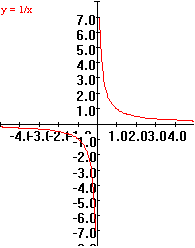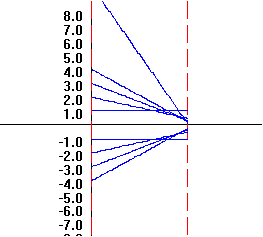Example 0.B.9   f(x) = 2x

 x f(x)=2x 2 4 1 2 0 1 -1 0.5 -2 0.25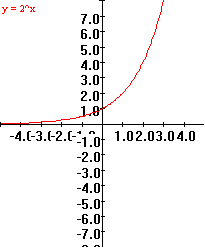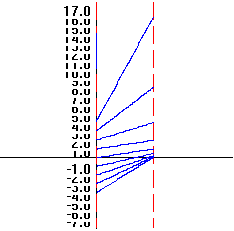Example 0.B.10 f(x) = 3

 x f(x)=3 2 3 1 3 0 3 -1 3 -2 3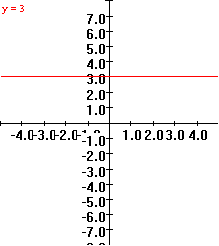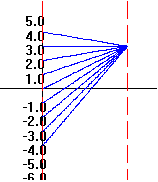Example 0.B.11 f(x) =-2x + 1

 x f(x)=-2x+1 2 -3 1 -1 0 1 -1 3 -2 5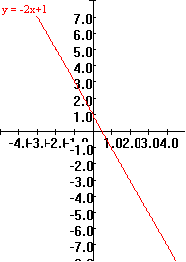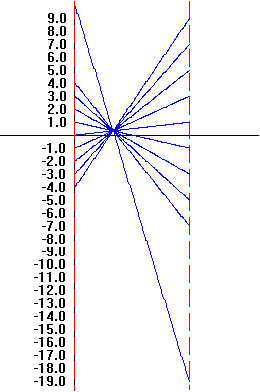Using GeoGebra to do this:
You can move the point on the x axis in the mapping diagram see the function values vary with the indicated value of x.
This point also moves the related point on the graph.
You can change the function definition for the graph and the mapping diagram.

 Martin Flashman, 26 June 2013, Created with GeoGebra

Review Exercises:
The exercises for this section cover material which you may recall from previous course work in algebra and trigonometry. You may not recall all of these topics or how to do the problems precisely. You may want to refer to the texts or notes from your previous courses in mathematics if you find these difficult. The skills needed to solve these problems will be important in the work ahead- so be careful to identify any difficulties you have with these problems and try to remedy any misunderstandings as you proceed.
1. For this problem let f be defined by `f (x) = 5x^2 + 3`. a) Find the following. Simplify you answer when possible.
i)   `f(1)`                 iii)  `f(1+h)`

ii)  `f(h)`                 iv) `{f(1+h) - f(1)\}/h`

b) Find any number(s) z where f(z) = 23.   c) For which values of x is f(x) < 23? Express your answer as an interval.

2. USING INTERVAL NOTATION, express the largest set of real numbers that can serve as the domain of each of the following functions:

a) `f(x)  =  (4 - x^2)/(x + 2)`         b) `g(x) =   1/(4-x^2)`

3. Suppose that F is defined by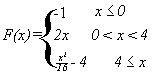.

Sketch a transformation figure and a complete graph of f.  Determine the domain and the range of f.

4. Solve for x:

a)  3 x-2 = 3 7-2x   b)  4 3x  = 8      c) 1/3(x - 5) = 2     d) 1/30 - 1/x = 1/6

5. Boyle's law states that, for a certain gas P*V = 320, where P is pressure and V is volume.

(a) Draw a complete graph representing this situation. Label your axes and write an equation for each asymptote.

(b) If `8 <= V <= 40`, what are the corresponding values of P?

6. Let f(x) = x 2 + 4x - 5. A. Find the axis of symmetry  and the vertex of f. B. Sketch a graph of f labeling clearly the coordinates of the vertex and the X- and Y- intercepts.

7. Old McDonald has a farm ,and on that farm she has some sheep and a pasture with a 200 meter long stone wall.  She wants to enclose a rectangular section of the pasture for a small sheep pen using the wall for one side and 140 meters of fencing she was given by her uncle Milo for the other three sides.

A. Let x denote the length of the fence that will be attached to the wall used as a side for the pen.  Which of the following equations express the area of the pen, A, as a function of x?
a. A = x ( 70 - x)                       b. A = 2 x ( 140 - x)
c. A = x ( 140 - x)                     d. A = x ( 200 - (1/2)x)
d. A = 2 x ( 70 - x)                    e. A = 2 x ( 200 - x)

17. Write a short story about cooking dinner. Discuss briefly those aspects of the meal's preparation that might be related by functions.

18. Write a short story about going shopping. Discuss briefly those aspects of the shopping that might be related by functions.

19. Write a short description of an ecological system. Discuss briefly those aspects of the system that might be related by functions.

20. Write a short description of the human body. Discuss briefly those aspects of the body that might be related by functions.

21. Write a short story about an athletic event or sports competition. Discuss briefly those aspects of the story that might be related by functions.